# Angle Sum Property of a Triangle:

There is a remarkable property connecting the three angles of a triangle.

• Draw a triangle. Cut on the three angles. Rearrange them as shown in the following Figure. The three angles now constitute one angle. This angle is a straight angle and so has measure 180°.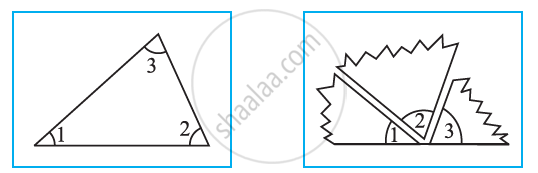Thus, the sum of the measures of the three angles of a triangle is 180°.

∴ ∠1 + ∠2 + ∠3 = 180°

• Take a piece of paper and cut out a triangle, say, ∆ABC.
Make the altitude AM by folding ∆ABC such that it passes through A.
Fold now the three corners such that all the three vertices A, B, and C touch at M.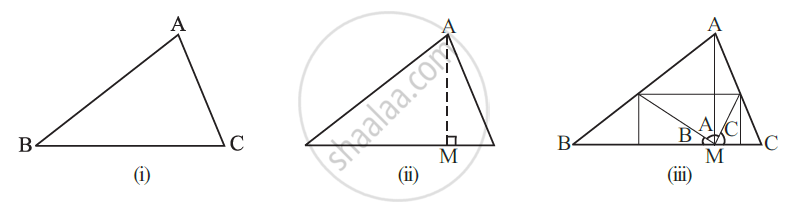You find that all the three angles form together a straight angle. This again shows that the sum of the measures of the three angles of a triangle is 180°.

∴ ∠B + ∠A + ∠C = 180°

# Angle Sum Property of a Triangle:

Theorem: The sum of the angles of a triangle is 180°.

Construction: Draw a line XPY parallel to QR through the opposite vertex P.

Proof: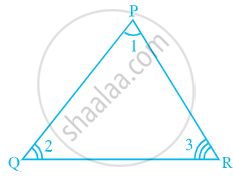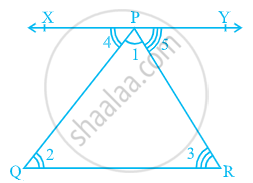In △ PQR,
Sum of all angles of a triangle is 180°.
∠PQR + ∠PRQ + ∠QPR = 180°......(1)

Since XY is a straight line, it can be concluded that:

Therefore, ∠XPY + ∠QRP + ∠RPY = 180°.

But XPY || QR and PQ, PR are transversals.

So,
∠XPY = ∠PQR.....(Pairs of alternate angles)

∠RPY = ∠PRQ.....(Pairs of alternate angles)

Substituting ∠XPY and ∠RPY in (1), we get

∠PQR + ∠PRQ + ∠QPR = 180°

Thus, The sum of the angles of a triangle is 180°.

## Example

In the given figure find m∠P.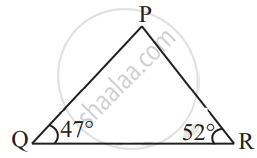By angle sum property of a triangle,
m∠P + 47° + 52° = 180°
Therefore,
m∠P = 180° – 47° – 52°
m∠P = 180° – 99°
m∠P = 81°

If you would like to contribute notes or other learning material, please submit them using the button below.

### Shaalaa.com

Angle Sum Property of a Triangle Theorem [00:05:30]
S
0%